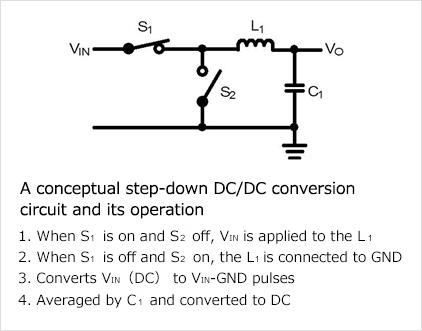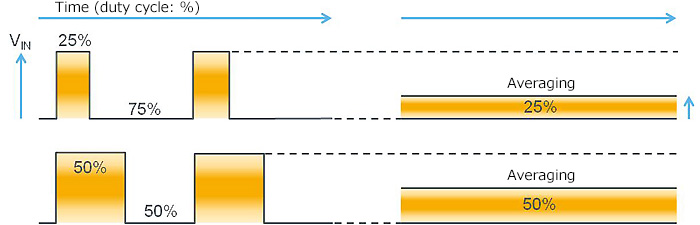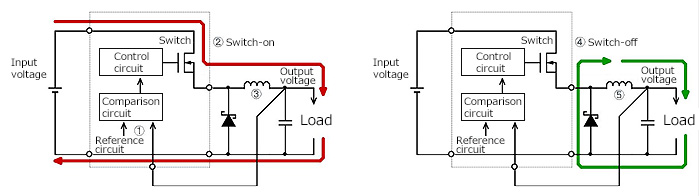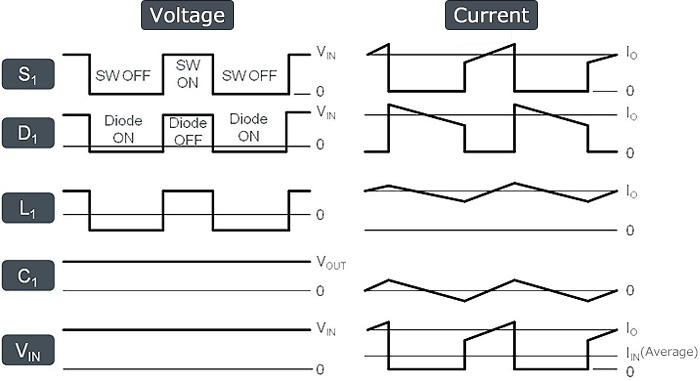Technical Information Site of Power Supply Design

• Basic Knowledge
• DC/DC
• Characteristics and Evaluation Method of Switching Regulators
• Switching Regulator Basics: Step-Down Operation Principles

2016.01.13 DC/DC

# Switching Regulator Basics: Step-Down Operation Principles

Characteristics and Evaluation Method of Switching RegulatorsIn what follows, we explain the operating principles of the switching regulator, taking as an example the step-down switching regulator, which is a regulator used most widely.

The step-down DC/DC conversion involves the time-division of a DC voltage (which is referred to as VIN) by means of a switch and smoothing of the results by means of an inductor and capacitor to produce a desired DC voltage. The figure on the right depicts a conceptual circuit for step-down DC/DC conversion and its operation.

In terms of PWM operation, a pulse period is specified so that with S1=ON and S2=OFF the duration of supply of VIN is 25%, and with S1=OFF and S2=ON, the duration of zero-volt (GND) state is 75%. When the obtained pulses are averaged, the result will be a 25% DC. Thus, if VIN is 10V, Vo, which is 25% of VIN will be 2.5V.In actual PWM, because the load current for the average output fluctuates, over a continuously fixed on-time, the voltage fluctuates as a function of the load current. Since such an operation would not rate as a regulator, when the output falls, the on-time is increased, and more energy is transmitted from the input side to elevate the output voltage. When the output voltage has restored itself sufficiently, the on-time is reduced to stop any further rise in output.

The circuit shown below represents an actual circuit replacing the concept diagram. The switch S1 is replaced with a MOSFET, and S2 with a Schottky diode. The figure also shows the comparison and control circuits that were omitted in the original diagram. This is a typical step-down switching circuit which is referred to as a non- synchronous or diode-rectifying circuit.Operation of a step-down switching regulator

1. Compares the output voltage with the reference voltage to determine if the former is equal to a set voltage
2. If the output voltage is lower than the set voltage, the switch is turned on, supplying power from the input to the output.
3. In this case, magnetic energy is stored in the inductor
4. The switch turns off when the output voltage exceeds the set voltage
5. The magnetic energy stored in the inductor is supplied to the output load, and it returns to the inductor.
6. The switch turns on again when the magnetic energy inductor is exhausted and the output voltage declines.

The switching waveforms for the actual current and voltage are shown below. S1 denotes a MOSFET switching transistor; D1, a Schottky diode; L1, an inductor; C1, an output voltage; and VIN, an input voltage.In this article, in the way of a review we explained the operation of a basic switching regulator. For actual evaluation, at each node the current and voltage waveform must be checked. Prerequisite to this exercise is a solid understanding of these basic operations.

#### Key Points:

・There are AC/DC and DC/DC switching regulators, each providing a variety of conversion methods.

・Selection of a method well-suited for a given design project requires a solid understanding of the pros and cons of each method.

・A key to understanding DC/DC conversion is that switching is employed to chop the energy necessary for the output in terms of time, and the results are rectified and smoothed for re-conversion to DC.

・Essential to evaluating the performance of a switching regulator is a good understanding of the current and voltage waveform in each node.